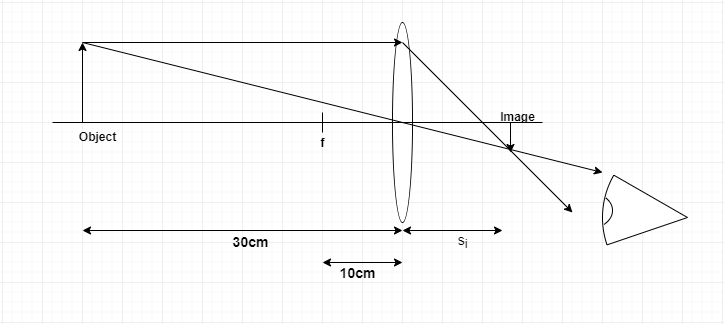# Problem: An object is 30 cm in front of a converging lens with a focal length of 10 cm. (a) Use ray tracing to determine the location of the image. (b What are the image characteristics: (i) upright or inverted, (ii) magnification, and (iii) real or virtual?

###### FREE Expert Solution

(a)

Thin lens equation:

$\overline{)\frac{\mathbf{1}}{{\mathbf{s}}_{\mathbf{o}}}{\mathbf{+}}\frac{\mathbf{1}}{{\mathbf{s}}_{\mathbf{i}}}{\mathbf{=}}\frac{\mathbf{1}}{\mathbf{f}}}$###### Problem Details

An object is 30 cm in front of a converging lens with a focal length of 10 cm.

(a) Use ray tracing to determine the location of the image.

(b What are the image characteristics: (i) upright or inverted, (ii) magnification, and (iii) real or virtual?